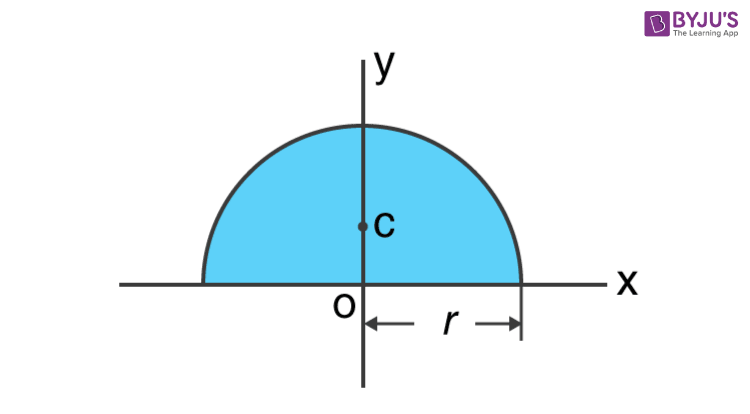Checkout JEE MAINS 2022 Question Paper Analysis : Checkout JEE MAINS 2022 Question Paper Analysis :

# Moment Of Inertia Of A Semicircle

Moment of inertia of a semicircle is generally expressed with the following equation;

 I = π R4 / 8

## How To Find The Moment Of Inertia Of A Semicircle

In order to find the moment of inertia of a semicircle, we need to recall the concept of deriving the moment of inertia of a circle. The concept can be used to easily determine the moment of inertia of a semicircle.

1. We will first begin with recalling the expression for a full circle.

I = πr4 / 4

In order to find the moment of inertia, we have to take the results of a full circle and basically divide it by two to get the result for a semicircle.

Now, in a full circle because of complete symmetry and area distribution, the moment of inertia relative to the x-axis is the same as the y-axis.

Ix = Iy = ¼ πr4

M.O.I relative to the origin, Jo = Ix + Iy = ¼ πr4 + ¼ πr4 = ½ πr4

Now we need to pull out the area of a circle which gives us;

Jo = ½ (πr2) R2

Similarly, for a semicircle, the moment of inertia of the x-axis is equal to the y-axis. Here, the semi-circle rotating about an axis is symmetric and therefore we consider the values equal. Here the M.O.I will be half the moment of inertia of a full circle. Now this gives us;

Ix = Iy = ⅛ πr4 = ⅛ (Ao) R2 = ⅛ (πr2) R2

Now to determine the semicircle’s moment of inertia we will take the sum of both the x and y-axis.

M.O.I relative to the origin, Jo = Ix + Iy = ⅛ πr4 + ⅛ πr4 = ¼ πr4

## Derivation

We will basically follow the polar coordinate method.The moment of inertia of the semicircle about the x-axis is

$$\begin{array}{l}I = \int y^{2}dA\end{array}$$
$$\begin{array}{l}\Rightarrow I_{x} = \int y^{2}dA\end{array}$$

y = r sin θ

dA = r drd θ

We will now determine the first moment of inertia about the x-axis. We get;

Ix = oroπ y2 ⋅ dA

oroπ (r sin θ) 2  . r drd θ

= oroπ r3 [(1 – cos2θ)/2](1/2) d2θdr

$$\begin{array}{l}I_{x} =\int_{0}^{r}\frac{r^{3}}{4}[2\theta -sin2\theta ]_{0}^{\pi }dr = \int_{0}^{r}\frac{r^{3}}{4}2\pi dr = \int_{0}^{r}\frac{r^{3}}{2}\pi dr\end{array}$$

$$\begin{array}{l}I_{x}=\left [ \frac{1}{8}\pi r^{4} \right ]_{0}^{r}= \frac{1}{8}\pi r^{4}\end{array}$$

We will now determine the first moment of inertia about the x-axis. We get;

Iy = oroπ x2 ⋅ dA

x = r cosθ

dA = r drd θ

Iyoroπ (r cos θ) 2  . r drd θ

= oroπ r3 [(1 + cos2θ)/2](1/2) d2θdr

$$\begin{array}{l}I_{x} =\int_{0}^{r}\frac{r^{3}}{4}[2\theta +sin2\theta ]_{0}^{\pi }dr = \int_{0}^{r}\frac{r^{3}}{4}2\pi dr = \int_{0}^{r}\frac{r^{3}}{2}\pi dr\end{array}$$

J0 = Ix + Iy

= (1/8) π r+(1/8) π r4

= (1/4) π r4

## Parallel Axis Theorem